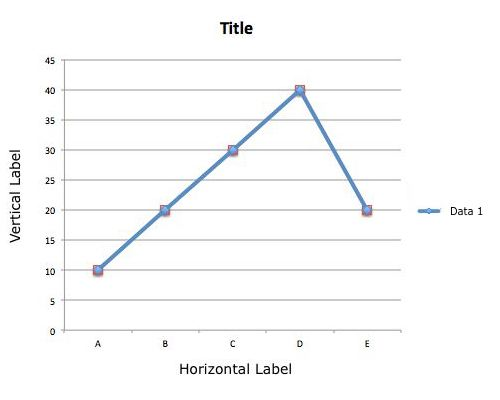DILR section has become a game changing section in the CAT. In the last two years this section has been the most difficult of all the three sections. Here is a DI set which will give a sneak peek at what can be expected in the CAT. This set comprises Type in the Answer(TITA) questions that have been a recent addition to the CAT.

Directions of question 1 to 4:

The investors can invest in four mutual funds – ICICI, SBI, Kotak and HDFC, to buy stocks of these four companies. Not all the investors invested in the mutual funds every month but when they did, each person invested Rs.100 to any one of the four mutual funds in a month, not necessarily the same fund every month.

For each of the four funds, the number of people who invested in each of October, November and December was either equal to or one greater or one lesser than their corresponding numbers in the previous month. For example, if ‘n’ investors invested in the ICICI fund in October, then the number of investors who invested in the ICICI fund in November can be ‘n – 1’, or ‘n’ or ‘n + 1’.Sitting arrangement is one of the popular topics of LRDI section for any competitive exam. The best thing about this topic is that you don’t need to apply any out of the box thinking but to process and write down all the information that is given to solve the questions. Sometimes you will feel that given information is not enough to complete the arrangements but remember your job is to solve the questions not to complete the arrangement.

Here I am giving you some sets of linear and circular arrangements, try to solve them and then match your answers with the given solution.

Direction for question number 1 to 4: Four couples A,B,C,D,E,F,G and H are sitting together on a bench facing towards north not necessarily in the same order. It is also known that

I. A,C,E,G are females who are sitting immediate left of their husbands.
II. G is sitting immediate right of H who is sitting three places away to the left of C.
III. A is sitting five places to the left of B who is sitting 3rd from extreme right.

[latexpage]
As the name suggests, data interpretation is all about logical interpretation of the given data and answering questions. Data interpretation carries the same weightage in third section of CAT as reading comprehension in verbal section or geometry and algebra in quant section. For the past two years (CAT 2014 & CAT 2015), CAT carries approximately 20 questions on data interpretation. If you haven’t been serious about this topic till now, there is never a better day than today to start!

Data can be represented in many ways, some of the most common ways are tables, bar graphs, Line Graphs, paragraphs, pie charts etc… This article will focus on LINE GRAPHS and will introduce you with the most basic aspects of a line graph.above is one of the basic example of a line graph and its various component are as follows:

Title : The title of the graph tells us what the graph is about
Label : Horizontal and vertical labels tell us about type of the data
Axis : X and Y axises tell us about the unit of the data.
Dots : Dots on the line graph tell us combination of X and Y axis data.
Line : The blue line connecting the dots in the above graph gives a visual representation of the data, and its variation.

[latexpage]In CAT and other MBA entrance exams, there are many questions which fall in the domain of equations (quadratic, cubic, quartic..) and the properties of their roots. Deriving these properties will not only help us remember them but also give us the basic concepts to solve all kinds of equations and their problems. In this chapter we shall learn how the properties of roots of a general polynomial equation are derived. So let’s begin!

Let’s take a polynomial P(x) in a single variable x:

Let \$P(x) = a_0(x)^n + a_1(x)^{n-1} + a_2(x)^{n-2} + … + a_{n-1}x + a_n\$ where \$a_1\$, \$a_2\$ etc. are constants (associated with decreasing powers of x) and n, n-1, etc. are the whole number powers of the variable x. The highest power of the variable x is known as the ‘degree’ of the polynomial P(x).

For example, \$P(x) = x^5 – 3x + 1\$ is a polynomial with degree 5 with \$a_0 = 1, a_1 = 0, a_2 = 0, a_3 = 0, a_4 = -3, a_5 = 1\$

• By madhusmita1, 2 months ago

• By Harshgarg12, 3 months ago

• Ohh Mmanymnay thanks dear for sharing previous papers, ...

By elyssarob65, 3 months ago

• Agree with comment above me.

By elyssarob65, 3 months ago

• median is the middle position Now when increase of 10 ...

By Gaurav Jain, 5 months ago

• product is an integer, hence x and y must take same val...

By Gaurav Jain, 5 months ago

• @vidisha-nagpal

By michaels1, 6 months ago

• In a circle chords ab and cd intersect perpendicularly ...

By tanvi, 7 months ago

• In the rectangle ABCD, the perpendicular bisector of AC...

By tanvi, 7 months ago

• By Sanjali, 7 months ago

• By Sanjali, 7 months ago

• Akbar & Birbal start together on a circular track o...

By Sanjali, 7 months ago